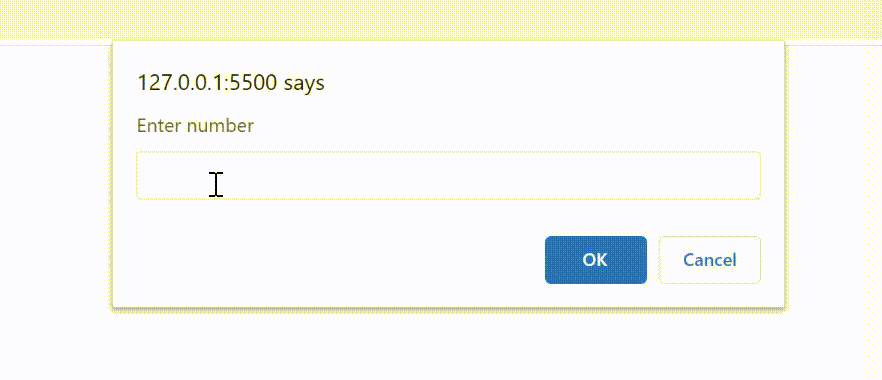Open in App
Not now

# How to create dynamic length array with numbers and sum the numbers using JavaScript ?

• Last Updated : 16 Jan, 2023

In JavaScript, array is a single variable that is used to store different elements. It is often used when we want to store a list of elements and access them by a single variable. Unlike most languages where the array is a reference to the multiple variables, in JavaScript array is a single variable that stores multiple elements.

JavaScript Arrays are Dynamic in nature which means, the length of the array can be modified at run time (when necessary). Array length can be specified after declaring the array as shown in the following example.

Example: This example shows the declaration of an array with length 10.

## Javascript

 `// Declare an array of undefined size``var` `arr = [];`` ` `// Set array length to 10``arr.length = 10;`` ` `// Print the length to console``console.log(arr);`

Output:

`Array(10) [ <10 empty slots> ]`

Example: As you can see, this method explains the array with 10 empty slots. The following example declares an array and takes input elements using a prompt dynamically and prints the sum of all elements and displays the result using an alert.

## Javascript

 `(``function` `() {``     ` `    ``// Declare an array of undefined size``    ``var` `arr = [];`` ` `    ``// Notice how the size of array``    ``// is not dot defined`` ` `    ``// Initiating loop to iterate``    ``// for inputs``    ``for` `(``var` `i = 0; i < 10; i++) { `` ` `        ``// Feeding integer inputs to array``        ``arr[i] = +prompt(``'Enter number'``);``         ` `        ``// Calculate sum of array elements``        ``var` `sum = arr.reduce(``function` `(a, b) {``            ``return` `a + b;``        ``}, 0)``    ``}``    ``alert(sum);``})();`

Output:

```Input : 1 2 3 4 5 6 7 8 9 10
Output :55```My Personal Notes arrow_drop_up